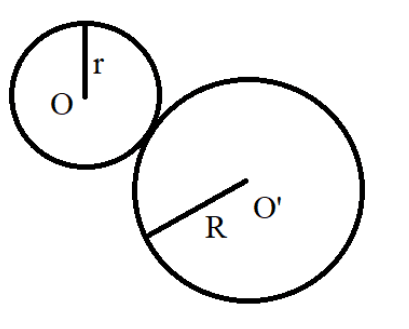Courses
Courses for Kids
Free study material
Offline Centres
MoreLast updated date: 30th Nov 2023
Total views: 280.2k
Views today: 6.80k

# Two circles touch externally. The sum of their area is $130\pi$ square centimetres and the distance between their centres is $14cm$. Find the radii of the circle.Verified
280.2k+ views
Hint: Here in this question, we are given two circles that touch each other. We are given that the sum of the area of the two circles is $130\pi$ square centimetres and the distance between the centres of the two circles is $14cm$. So, we have to find the radii of the two circles in the problem. We know that the formula for finding the area of a circle, we have a standard formula is $A = \pi {r^2}$ where r is the radius of the circle.

So, there are two touching circles whose sum of area is $130\pi$ square centimetres.
Let the radius of the one circle be r and the radius of the other circle be R.Hence, we have,
$\pi {r^2} + \pi {R^2} = 130\pi \;c{m^2}$
Dividing both the sides of the equation by $\pi$, we get,
$\Rightarrow {r^2} + {R^2} = 130\;c{m^2} - - - - - \left( 1 \right)$
Also, the distance between the centres of the two circles is $14cm$. So, we get,
$\Rightarrow r + R = 14cm - - - - - \left( 2 \right)$
Squaring both sides of the above equation, we get,
$\Rightarrow {\left( {r + R} \right)^2} = {\left( {14cm} \right)^2}$
Evaluating the square of the left side of the equation, we get,
$\Rightarrow {r^2} + 2Rr + {R^2} = 196\;c{m^2}$
Now, substituting the value of $\left( {{r^2} + {R^2}} \right)$ from equation $\left( 1 \right)$, we get,
$\Rightarrow 2Rr = 196\;c{m^2} - 130\;c{m^2}$
Simplifying the equation further, we get,
$\Rightarrow 2Rr = 66\;c{m^2}$
$\Rightarrow Rr = 33\;c{m^2} - - - - - \left( 3 \right)$
Now, we can solve for the radii of both the circles using the equation $\left( 2 \right)$ and $\left( 3 \right)$.
So, substituting the value of R from the equation $\left( 2 \right)$ in equation $\left( 3 \right)$, we get,
$\Rightarrow \left( {14cm - r} \right)r = 33\;c{m^2}$
Now, we open the brackets,
$\Rightarrow 14r - {r^2} = 33$
Shifting all the terms of the equation to the right side of the equation, we get,
$\Rightarrow {r^2} - 14r + 33 = 0$
Now, we have to solve this quadratic equation in r so as to find the radii of both the circles. We solve the quadratic equation using splitting the middle term method.
We split the middle term of the quadratic equation into two parts and factorise the equation further.
$\Rightarrow {r^2} - 11r - 3r + 33 = 0$
Taking out r common from the first two terms,
$\Rightarrow r\left( {r - 11} \right) - 3\left( {r - 11} \right) = 0$
Now, taking out the factor $\left( {r - 11} \right)$ common, we get,
$\Rightarrow \left( {r - 11} \right)\left( {r - 3} \right) = 0$
Now, either $\left( {r - 3} \right) = 0$ or $\left( {r - 11} \right) = 0$
$\Rightarrow r = 3$ or $r = 11$
Hence, the value of r is $3$ or $11$.
Now, we know that $r + R = 14cm$
If the value of r is $3$, then the value of R is $11$.
If the value of r is $11$, then the value of R is $3$.
Hence, the radii of the circle are $3\;cm$ and $11\;cm$.

Note: A circle is a closed two dimensional figure. The area of a circle is defined as the region occupied by the circular region. It can be determined by using formula $A = \pi {r^2}$ where r is the radius of the circle. The radius is denoted by r or R. Quadratic equations are the polynomial equations with degree of the variable or unknown as $2$. Quadratic equations can be solved by splitting the middle term, using the quadratic formula and completing the square method. Some equations don’t appear to be quadratic but can be reduced into quadratic equations using substitution.